# RD Sharma Solutions for Class 10 Maths Chapter 5 Trigonometric Ratios

## RD Sharma Solutions Class 10 Maths Chapter 5 – Free PDF Download

RD Sharma Solutions for Class 10 Maths Chapter 5 – Trigonometric Ratios are provided here for students to grasp the concepts in depth. A proper guidance tool in the right direction is essential for a student to excel in exams. The RD Sharma Solutions are resources prepared by our experts at BYJU’S following the latest CBSE guidelines. This will definitely help the students secure high marks in their examinations, as the solutions are designed in an easily understandable language.

The PDF of RD Sharma Solutions Class 10 Chapter 5 Trigonometric Ratios are provided here. The textbook questions have been solved by BYJU’S experts in Maths with the intention to  help students solve the problems without any difficulties. By following RD Sharma Solutions for Class 10, students can obtain worthy results in the board exams.

Trigonometry is the Science of measuring triangles. Further, in this chapter, you will learn about the trigonometric ratios and their relations between them. In addition, the Trigonometric Ratios of some specific angles are also discussed.

## RD Sharma Solutions for Class 10 Maths Chapter 5 Trigonometric Ratios

### RD Sharma Solutions for Class 10 Maths Chapter 5 Exercise 5.1 Page No: 5.23

1. In each of the following, one of the six trigonometric ratios s given. Find the values of the other trigonometric ratios.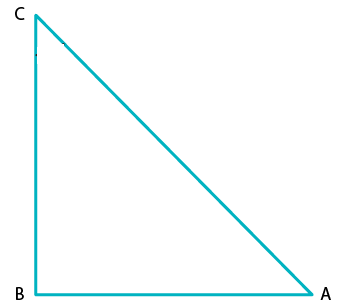(i) sin A = 2/3

Solution:

We have,

sin A = 2/3 ……..….. (1)

As we know, by sin definition,

sin A =  Perpendicular/ Hypotenuse = 2/3 ….(2)

By comparing eq. (1) and (2), we have

Opposite side = 2 and Hypotenuse = 3

Now, on using Pythagoras theorem in Δ ABC

AC= AB2 + BC2

Putting the values of perpendicular side (BC) and hypotenuse (AC) and for the base side as (AB), we get

⇒ 32 = AB2 + 22

AB= 32 – 22

AB2 = 9 – 4

AB2 = 5

AB = √5

Hence, Base = √5

By definition,

cos A = Base/Hypotenuse

⇒ cos A = √5/3

Since, cosec A = 1/sin A = Hypotenuse/Perpendicular

⇒ cosec A = 3/2

And, sec A = Hypotenuse/Base

⇒ sec A = 3/√5

And, tan A = Perpendicular/Base

⇒ tan A =  2/√5

And, cot A = 1/ tan A = Base/Perpendicular

⇒ cot A = √5/2

(ii) cos A = 4/5

Solution:

We have,

cos A = 4/5 …….…. (1)

As we know, by cos definition,

cos A = Base/Hypotenuse …. (2)

By comparing eq. (1) and (2), we get

Base = 4 and Hypotenuse = 5

Now, using Pythagoras’ theorem in Δ ABC,

AC= AB+ BC2

Putting the value of base (AB) and hypotenuse (AC) and for the perpendicular (BC), we get

52 = 42 + BC2

BC2 = 52 – 42

BC2 = 25 – 16

BC2 = 9

BC= 3

Hence, Perpendicular = 3

By definition,

sin A = Perpendicular/Hypotenuse

⇒ sin A = 3/5

Then, cosec A = 1/sin A

⇒ cosec A= 1/ (3/5) = 5/3 = Hypotenuse/Perpendicular

And, sec A = 1/cos A

⇒ sec A =Hypotenuse/Base

sec A = 5/4

And, tan A = Perpendicular/Base

⇒ tan A = 3/4

Next, cot A = 1/tan A = Base/Perpendicular

∴ cot A = 4/3

(iii) tan θ = 11/1

Solution:

We have, tan θ = 11…..…. (1)

By definition,

tan θ = Perpendicular/ Base…. (2)

On Comparing eq. (1) and (2), we get;

Base = 1 and Perpendicular = 5

Now, using Pythagoras’ theorem in Δ ABC,

AC2 = AB2 + BC2

Putting the value of base (AB) and perpendicular (BC) to get hypotenuse(AC), we get

AC2 = 12 + 112

AC2 = 1 + 121

AC2= 122

AC= √122

Hence, hypotenuse = √122

By definition,

sin = Perpendicular/Hypotenuse

⇒ sin θ = 11/√122

And, cosec θ = 1/sin θ

⇒ cosec θ = √122/11

Next, cos θ = Base/ Hypotenuse

⇒ cos θ = 1/√122

And, sec θ = 1/cos θ

⇒ sec θ = √122/1 = √122

And, cot θ  = 1/tan θ

∴ cot θ = 1/11

(iv) sin θ = 11/15

Solution:

We have,  sin θ = 11/15 ………. (1)

By definition,

sin θ = Perpendicular/ Hypotenuse …. (2)

On Comparing eq. (1) and (2), we get,

Perpendicular = 11 and Hypotenuse= 15

Now, using Pythagoras’ theorem in Δ ABC,

AC2 = AB2 + BC2

Putting the value of perpendicular (BC) and hypotenuse (AC) to get the base (AB), we have

152 = AB2 +112

AB2 = 15– 112

AB2 = 225 – 121

AB= 104

AB = √104

AB= √ (2×2×2×13)

AB= 2√(2×13)

AB= 2√26

Hence, Base = 2√26

By definition,

cos θ = Base/Hypotenuse

∴ cosθ = 2√26/ 15

And, cosec θ = 1/sin θ

∴ cosec θ = 15/11

And, secθ = Hypotenuse/Base

∴ secθ =15/ 2√26

And,  tan θ = Perpendicular/Base

∴ tanθ =11/ 2√26

And,  cot θ = Base/Perpendicular

∴ cotθ =2√26/ 11

(v) tan α = 5/12

Solution:

We have,  tan α = 5/12 …. (1)

By definition,

tan α = Perpendicular/Base…. (2)

On Comparing eq. (1) and (2), we get

Base = 12 and Perpendicular side = 5

Now, using Pythagoras’ theorem in Δ ABC,

AC2 = AB2 + BC2

Putting the value of base (AB) and the perpendicular (BC) to get hypotenuse (AC), we have

AC2 = 122 + 52

AC2 = 144 + 25

AC2= 169

AC = 13 [After taking sq root on both sides]

Hence, Hypotenuse = 13

By definition,

sin α  = Perpendicular/Hypotenuse

∴ sin α = 5/13

And, cosec α  = Hypotenuse/Perpendicular

∴ cosec α = 13/5

And,  cos α = Base/Hypotenuse

∴ cos α = 12/13

And,  sec α =1/cos α

∴ sec α = 13/12

And, tan α = sin α/cos α

∴ tan α=5/12

Since, cot α = 1/tan α

∴ cot α =12/5

(vi) sin θ = √3/2

Solution:

We have, sin θ = √3/2 …………. (1)

By definition,

sin θ = Perpendicular/ Hypotenuse….(2)

On Comparing eq. (1) and (2), we get

Perpendicular = √3 and Hypotenuse = 2

Now, using Pythagoras’ theorem in Δ ABC,

AC2 = AB2 + BC2

Putting the value of perpendicular (BC) and hypotenuse (AC) and get the base (AB), we get

22 = AB2 + (√3)2

AB2 = 22 – (√3)2

AB2 = 4 – 3

AB2 = 1

AB = 1

Thus, Base = 1

By definition,

cos θ = Base/Hypotenuse

∴ cos θ = 1/2

And, cosec θ = 1/sin θ

Or cosec θ= Hypotenuse/Perpendicualar

∴ cosec θ =2/√3

And,  sec θ = Hypotenuse/Base

∴ sec θ = 2/1

And,  tan θ = Perpendicula/Base

∴ tan θ = √3/1

And,  cot θ = Base/Perpendicular

∴ cot θ = 1/√3

(vii) cos θ = 7/25

Solution:

We have, cos θ = 7/25 ……….. (1)

By definition,

cos θ = Base/Hypotenuse

On Comparing eq. (1) and (2), we get;

Base = 7 and Hypotenuse = 25

Now, using Pythagoras’ theorem in Δ ABC,

AC2= AB2 + BC2

Putting the value of base (AB) and hypotenuse (AC) to get the perpendicular (BC),

252 = 7+BC2

BC2 = 252 – 72

BC2 = 625 – 49

BC2 = 576

BC= √576

BC= 24

Hence, Perpendicular side = 24

By definition,

sin θ = perpendicular/Hypotenuse

∴ sin θ = 24/25

Since, cosec θ = 1/sin θ

Also, cosec θ= Hypotenuse/Perpendicualar

∴ cosec θ = 25/24

Since,  sec θ = 1/cosec θ

Also,  sec θ = Hypotenuse/Base

∴ sec θ = 25/7

Since,  tan θ = Perpendicular/Base

Now,  cot = 1/tan θ

So,  cot θ = Base/Perpendicular

∴ cot θ = 7/24

(viii) tan θ = 8/15

Solution:

We have, tan θ = 8/15 …………. (1)

By definition,

tan θ = Perpendicular/Base …. (2)

On Comparing eq. (1) and (2), we get

Base = 15 and Perpendicular = 8

Now, using Pythagoras’ theorem in Δ ABC,

AC2 = 152 + 82

AC2 = 225 + 64

AC2 = 289

AC = √289

AC = 17

Hence, Hypotenuse = 17

By definition,

Since,  sin θ = perpendicular/Hypotenuse

∴ sin θ = 8/17

Since, cosec θ = 1/sin θ

Also, cosec θ = Hypotenuse/Perpendicular

∴ cosec θ = 17/8

Since,  cos θ = Base/Hypotenuse

∴ cos θ = 15/17

Since,  sec θ = 1/cos θ

Also,  sec θ = Hypotenuse/Base

∴ sec θ = 17/15

Since, cot θ = 1/tan θ

Also,  cot θ = Base/Perpendicular

∴ cot θ = 15/8

(ix) cot θ = 12/5

Solution:

We have, cot θ = 12/5 …………. (1)

By definition,

cot θ = 1/tan θ

cot θ = Base/Perpendicular ……. (2)

On Comparing eq. (1) and (2), we have

Base = 12 and Perpendicular side = 5

Now, using Pythagoras’ theorem in Δ ABC,

AC2= AB2 + BC2

Putting the value of base (AB) and perpendicular (BC) to get the hypotenuse (AC),

AC2 = 122 + 52

AC2= 144 + 25

AC2 = 169

AC = √169

AC = 13

Hence, Hypotenuse = 13

By definition,

Since,  sin θ = perpendicular/Hypotenuse

∴ sin θ= 5/13

Since, cosec θ = 1/sin θ

Also, cosec θ= Hypotenuse/Perpendicualar

∴ cosec θ = 13/5

Since,  cos θ = Base/Hypotenuse

∴ cos θ = 12/13

Since,  sec θ = 1/cosθ

Also,  sec θ = Hypotenuse/Base

∴ sec θ = 13/12

Since,  tanθ = 1/cot θ

Also,  tan θ = Perpendicular/Base

∴ tan θ = 5/12

(x)  sec θ = 13/5

Solution:

We have, sec θ = 13/5…….… (1)

By definition,

sec θ = Hypotenuse/Base…………. (2)

On Comparing eq. (1) and (2), we get

Base = 5 and Hypotenuse = 13

Now, using Pythagoras’ theorem in Δ ABC,

AC= AB2 + BC2

And putting the value of base side (AB) and hypotenuse (AC) to get the perpendicular side (BC),

132 = 52 + BC2

BC2 = 13– 52

BC2=169 – 25

BC2= 144

BC= √144

BC = 12

Hence, Perpendicular = 12

By definition,

Since,  sin θ = perpendicular/Hypotenuse

∴ sin θ= 12/13

Since, cosec θ= 1/ sin θ

Also, cosec θ= Hypotenuse/Perpendicular

∴ cosec θ = 13/12

Since,  cos θ= 1/sec θ

Also,  cos θ = Base/Hypotenuse

∴ cos θ = 5/13

Since,  tan θ = Perpendicular/Base

∴ tan θ = 12/5

Since,  cot θ = 1/tan θ

Also,  cot θ = Base/Perpendicular

∴ cot θ = 5/12

(xi)  cosec θ = √10

Solution:

We have, cosec θ = √10/1   ……..… (1)

By definition,

cosec θ = Hypotenuse/ Perpendicualar …….….(2)

And, cosecθ = 1/sin θ

On comparing eq.(1) and(2), we get

Perpendicular side = 1 and Hypotenuse = √10

Now, using Pythagoras’ theorem in Δ ABC,

AC= AB2 + BC2

Putting the value of perpendicular (BC) and hypotenuse (AC) to get the base side (AB),

(√10)2 = AB2 + 12

AB2= (√10)2 – 12

AB2= 10 – 1

AB = √9

AB = 3

So, Base side = 3

By definition,

Since,  sin θ = Perpendicular/Hypotenuse

∴ sin θ = 1/√10

Since,  cos θ = Base/Hypotenuse

∴ cos θ = 3/√10

Since,  sec θ = 1/cos θ

Also, sec θ = Hypotenuse/Base

∴ sec θ = √10/3

Since,  tan θ = Perpendicular/Base

∴ tan θ = 1/3

Since,  cot θ = 1/tan θ

∴ cot θ = 3/1

(xii)  cos θ =12/15

Solution:

We have; cos θ = 12/15 ………. (1)

By definition,

cos θ = Base/Hypotenuse……… (2)

By comparing eq. (1) and (2), we get;

Base =12 and Hypotenuse = 15

Now, using Pythagoras’ theorem in Δ ABC, we get

AC2 = AB2+ BC2

Putting the value of base (AB) and hypotenuse (AC) to get the perpendicular (BC),

152 = 122 + BC2

BC2 = 152 – 122

BC2 = 225 – 144

BC 2= 81

BC = √81

BC = 9

So, Perpendicular = 9

By definition,

Since,  sin θ = perpendicular/Hypotenuse

∴ sin θ = 9/15 = 3/5

Since, cosec θ = 1/sin θ

Also, cosec θ = Hypotenuse/Perpendicular

∴ cosec θ= 15/9 = 5/3

Since,  sec θ = 1/cos θ

Also,  sec θ = Hypotenuse/Base

∴ sec θ = 15/12 = 5/4

Since,  tan θ = Perpendicular/Base

∴ tan θ = 9/12 = 3/4

Since,  cot θ = 1/tan θ

Also,  cot θ = Base/Perpendicular

∴ cot θ = 12/9 = 4/3

2. In a △ ABC, right-angled at B, AB = 24 cm , BC = 7 cm. Determine

(i) sin A , cos A (ii) sin C, cos C

Solution: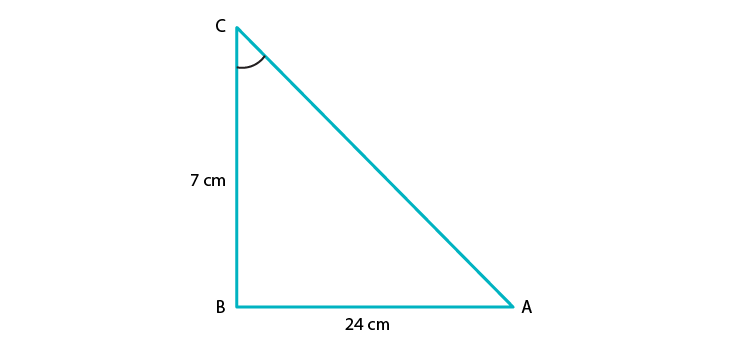(i) Given: In △ABC, AB = 24 cm, BC = 7cm and ∠ABC = 90o

To find: sin A, cos A

By using Pythagoras’ theorem in △ABC, we have

AC2 = AB2 + BC2

AC2 = 242 + 72

AC2 = 576 + 49

AC2= 625

AC = √625

AC= 25

Hence, Hypotenuse = 25

By definition,

sin A = Perpendicular side opposite to angle A/ Hypotenuse

sin A = BC/ AC

sin A = 7/ 25

And,

cos A = Base side adjacent to angle A/Hypotenuse

cos A = AB/ AC

cos A = 24/ 25

(ii) Given: In △ABC , AB = 24 cm and BC = 7cm and ∠ABC = 90o

To find: sin C, cos C

By using Pythagoras’ theorem in △ABC, we have

AC2 = AB2 + BC2

AC2 = 242 + 72

AC2 = 576 + 49

AC2= 625

AC = √625

AC= 25

Hence, Hypotenuse = 25

By definition,

sin C = Perpendicular side opposite to angle C/Hypotenuse

sin C = AB/ AC

sin C = 24/ 25

And,

cos C = Base side adjacent to angle C/Hypotenuse

cos A = BC/AC

cos A = 7/25

3. In fig. 5.37, find tan P and cot R. Is tan P = cot R?

Solution: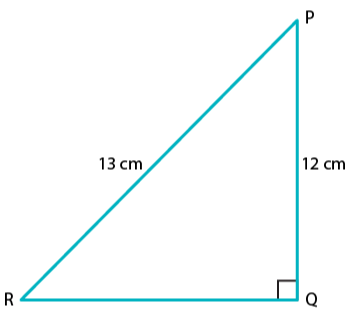By using Pythagoras theorem in △PQR, we have

PR2 = PQ2 + QR2

Putting the length of given side PR and PQ in the above equation,

132 = 122 + QR2

QR2 = 132 – 122

QR2 = 169 – 144

QR2 = 25

QR = √25 = 5

By definition,

tan P = Perpendicular side opposite to P/ Base side adjacent to angle P

tan P = QR/PQ

tan P = 5/12 ………. (1)

And,

cot R= Base/Perpendicular

cot R= QR/PQ

cot R= 5/12 …. (2)

When comparing equation (1) and (2), we can see that R.H.S of both the equation is equal.

Therefore, L.H.S of both equations should also be equal.

∴ tan P = cot R

Yes, tan P = cot R = 5/12

4. If sin A = 9/41, compute cos A and tan A.

Solution: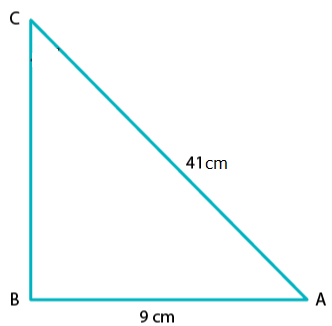Given that, sin A = 9/41 …………. (1)

Required to find: cos A, tan A

By definition, we know that

sin A = Perpendicular/ Hypotenuse……………(2)

On Comparing eq. (1) and (2), we get

Perpendicular side = 9 and Hypotenuse = 41

Let’s construct △ABC as shown below,

And, here the length of base AB is unknown.

Thus, by using Pythagoras theorem in △ABC, we get

AC2 = AB2 + BC2

412 = AB2 + 92

AB2 = 412 – 92

AB2 = 168 – 81

AB= 1600

AB = √1600

AB = 40

⇒ Base of triangle ABC, AB = 40

We know that,

cos A = Base/ Hypotenuse

cos A =AB/AC

cos A =40/41

And,

tan A = Perpendicular/ Base

tan A = BC/AB

tan A = 9/40

5.  Given 15cot A= 8, find sin A and sec A.

Solution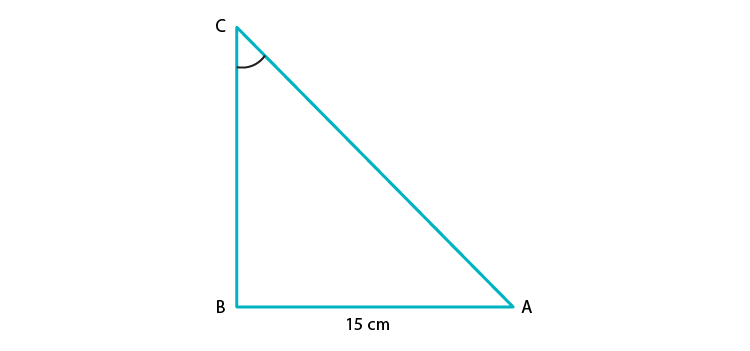We have, 15cot A = 8

Required to find: sin A and sec A

As, 15 cot A = 8

⇒ cot A = 8/15 …….(1)

And we know,

cot A = 1/tan A

Also by definition,

Cot A = Base side adjacent to ∠A/ Perpendicular side opposite to ∠A …. (2)

On comparing equation (1) and (2), we get

Base side adjacent to ∠A = 8

Perpendicular side opposite to ∠A = 15

So, by using Pythagoras theorem to △ABC, we have

AC2 = AB2 +BC2

Substituting values for sides from the figure

AC2 = 82 + 152

AC2 = 64 + 225

AC2 = 289

AC = √289

AC = 17

Therefore, hypotenuse =17

By definition,

sin A = Perpendicular/Hypotenuse

⇒ sin A= BC/AC

sin A= 15/17 (using values from the above)

Also,

sec A= 1/ cos A

⇒ secA = Hypotenuse/ Base side adjacent to ∠A

∴ sec A= 17/8

6. In △PQR, right-angled at Q, PQ = 4cm and RQ = 3 cm. Find the value of sin P, sin R, sec P and sec R.

Solution: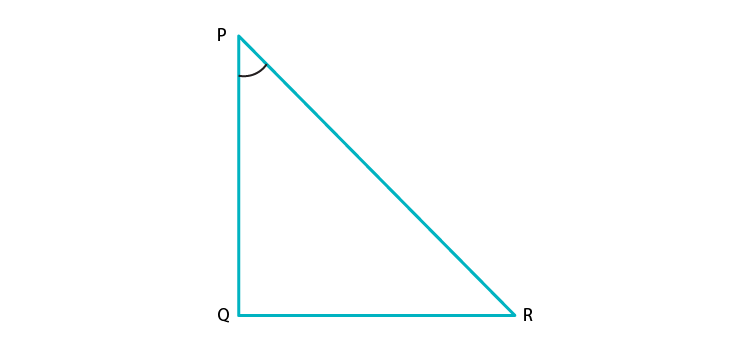Given:

△PQR is right-angled at Q.

PQ = 4cm

RQ = 3cm

Required to find: sin P, sin R, sec P, sec R

Given △PQR,

By using Pythagoras theorem to △PQR, we get

PR2 = PQ2 +RQ2

Substituting the respective values,

PR2 = 42 +32

PR2 = 16 + 9

PR2 = 25

PR = √25

PR = 5

⇒ Hypotenuse =5

By definition,

sin P = Perpendicular side opposite to angle P/ Hypotenuse

sin P = RQ/ PR

⇒ sin P = 3/5

And,

sin R = Perpendicular side opposite to angle R/ Hypotenuse

sin R = PQ/ PR

⇒ sin R = 4/5

And,

sec P=1/cos P

secP = Hypotenuse/ Base side adjacent to ∠P

sec P = PR/ PQ

⇒ sec P = 5/4

Now,

sec R = 1/cos R

secR = Hypotenuse/ Base side adjacent to ∠R

sec R = PR/ RQ

⇒ sec R = 5/3

7. If cot θ = 7/8, evaluate

(i)   (1+sin θ)(1–sin θ)/ (1+cos θ)(1–cos θ)

(ii)  cot2 θ

Solution:

(i) Required to evaluate: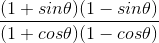, given = cot θ = 7/8

Taking the numerator, we have

(1+sin θ)(1–sin θ) = 1 – sin2 θ [Since, (a+b)(a-b) = a2 – b2]

Similarly,

(1+cos θ)(1–cos θ) = 1 – cos2 θ

We know that,

sin2 θ + cos2 θ = 1

⇒ 1 – cos2 θ = sin2 θ

And,

1 – sin2 θ = cos2 θ

Thus,

(1+sin θ)(1 –sin θ) = 1 – sin2 θ = cos2 θ

(1+cos θ)(1–cos θ) = 1 – cos2 θ = sin2 θ= cos2 θ/ sin2 θ

= (cos θ/sin θ)2

And, we know that (cos θ/sin θ) = cot θ= (cot θ)2

= (7/8)2

= 49/ 64

(ii) Given,

cot θ = 7/8

So, by squaring on both sides we get

(cot θ)2 = (7/8)2

∴ cot θ2 = 49/64

8. If 3cot A = 4, check whether (1–tan2A)/(1+tan2A) = (cos2A – sin2A) or not.

Solution: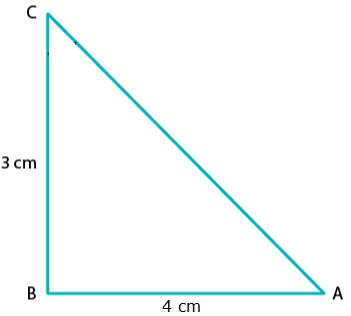Given,

3cot A = 4

⇒ cot A = 4/3

By definition,

tan A = 1/ Cot A = 1/ (4/3)

⇒ tan A = 3/4

Thus,

Base side adjacent to ∠A = 4

Perpendicular side opposite to ∠A = 3

In ΔABC, Hypotenuse is unknown.

Thus, by applying Pythagoras theorem in ΔABC,

We get

AC= AB2 + BC2

AC2 = 42 + 32

AC2 = 16 + 9

AC2 = 25

AC = √25

AC = 5

Hence, hypotenuse = 5

Now, we can find that

sin A = opposite side to ∠A/ Hypotenuse = 3/5

And,

cos A = adjacent side to ∠A/ Hypotenuse = 4/5

Taking the LHS,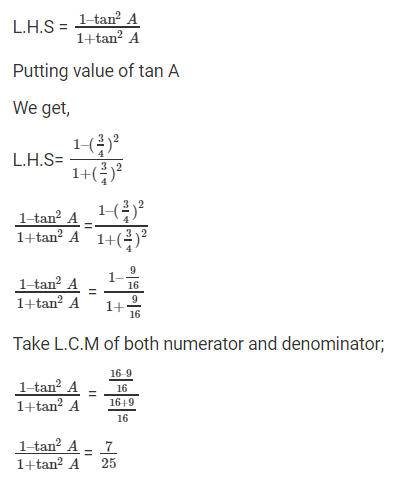Thus, LHS = 7/25

Now, taking RHS,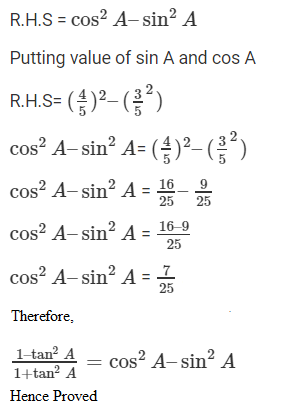9. If tan θ = a/b, find the value of (cos θ + sin θ)/ (cos θ – sin θ)

Solution:

Given,

tan θ = a/b

And we know by definition that

tan θ = opposite side/ adjacent side

Thus, by comparison,

Opposite side = a and adjacent side = b

To find the hypotenuse, we know that by Pythagoras theorem that

Hypotenuse2 = opposite side2 + adjacent side2

⇒ Hypotenuse = √(a2 + b2)

So, by definition

sin θ = opposite side/ Hypotenuse

sin θ = a/ √(a2 + b2)

And,

cos θ = adjacent side/ Hypotenuse

cos θ = b/ √(a2 + b2)

Now,

After substituting for cos θ and sin θ, we have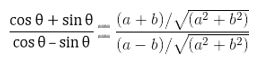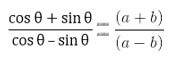Hence, proved.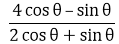10. If 3 tan θ = 4, find the value of

Solution:

Given, 3 tan θ = 4

⇒ tan θ = 4/3From, let’s divide the numerator and denominator by cos θ.

We get,

(4 – tan θ) / (2 + tan θ)

⇒ (4 – (4/3)) / (2 + (4/3)) [using the value of tan θ]

⇒ (12 – 4) / (6 + 4) [After taking LCM and cancelling it]

⇒ 8/10 = 4/5∴ = 4/5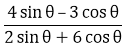11. If 3 cot θ = 2, find the value of

Solution:

Given, 3 cot θ = 2

⇒ cot θ = 2/3From, let’s divide the numerator and denominator by sin θ.

We get,

(4 –3 cot θ) / (2 + 6 cot θ)

⇒ (4 – 3(2/3)) / (2 + 6(2/3)) [using the value of tan θ]

⇒ (4 – 2) / (2 + 4) [After taking LCM and simplifying it]

⇒ 2/6 = 1/3∴ = 1/3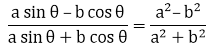12. If tan θ = a/b, prove that

Solution:

Given, tan θ = a/b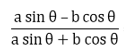From LHS, let’s divide the numerator and denominator by cos θ.

And we get,

(a tan θ – b) / (a tan θ + b)

⇒ (a(a/b) – b) / (a(a/b) + b) [using the value of tan θ]

⇒ (a2 – b2)/b2 / (a2 + b2)/b2 [After taking LCM and simplifying it]

⇒ (a2 – b2)/ (a2 + b2)

= RHS

Hence, proved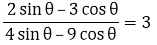13. If sec θ = 13/5, show that

Solution: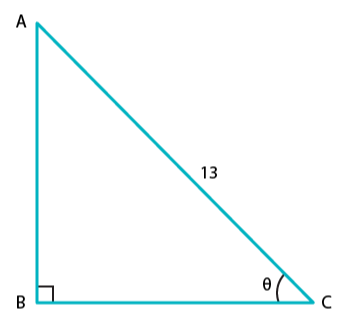Given,

sec θ = 13/5

We know that,

sec θ = 1/ cos θ

⇒ cos θ = 1/ sec θ = 1/ (13/5)

∴ cos θ = 5/13 ……. (1)

By definition,

cos θ = adjacent side/ hypotenuse ….. (2)

Comparing (1) and (2), we have

Adjacent side = 5 and hypotenuse = 13

By Pythagoras theorem,

Opposite side = √((hypotenuse) 2 – (adjacent side)2)

= √(132 – 52)

= √(169 – 25)

= √(144)

= 12

Thus, the opposite side = 12

By definition,

tan θ = opposite side/ adjacent side

∴ tan θ = 12/ 5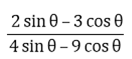From, let’s divide the numerator and denominator by cos θ.

We get,

(2 tan θ – 3) / (4 tan θ – 9)

⇒ (2(12/5) – 3) / (4(12/5) – 9) [using the value of tan θ]

⇒ (24 – 15) / (48 – 45) [After taking LCM and cancelling it]

⇒ 9/3 = 3∴ = 3

14. If cos θ = 12/13, show that sin θ(1 – tan θ) = 35/156

Solution: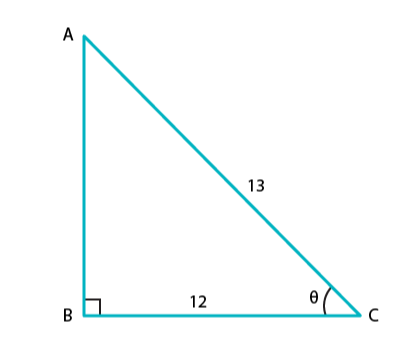Given, cos θ = 12/13…… (1)

By definition, we know that

cos θ = Base side adjacent to ∠θ / Hypotenuse……. (2)

When comparing equation (1) and (2), we get

Base side adjacent to ∠θ = 12 and Hypotenuse = 13

From the figure,

Base side BC = 12

Hypotenuse AC = 13

Side AB is unknown here, and it can be found by using Pythagoras theorem.

Thus, by applying Pythagoras theorem,

AC2 = AB2 + BC2

132 = AB2 + 122

Therefore,

AB2 = 13– 122

AB2 = 169 – 144

AB2 = 25

AB = √25

AB = 5 …. (3)

Now, we know that

sin θ = Perpendicular side opposite to ∠θ / Hypotenuse

Thus, sin θ = AB/AC [from figure]

⇒ sin θ = 5/13… (4)

And, tan θ = sin θ / cos θ = (5/13) / (12/13)

⇒ tan θ = 12/13… (5)

Taking L.H.S we have

L.H.S = sin θ (1 – tan θ)

Substituting the value of sin θ and tan θ from equation (4) and (5),

We get,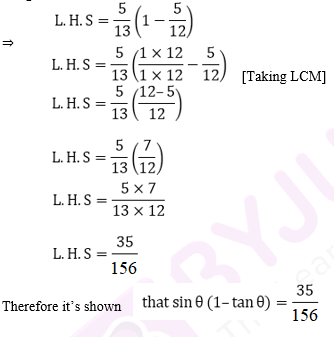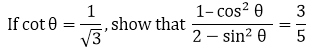15.

Solution: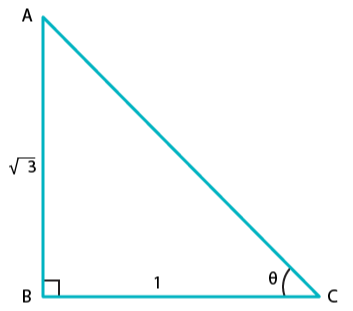Given, cot θ = 1/3……. (1)

By definition, we know that,

cot θ = 1/ tan θ

And, since tan θ = perpendicular side opposite to ∠θ / Base side adjacent to ∠θ

⇒ cot θ = Base side adjacent to ∠θ / perpendicular side opposite to ∠θ …… (2)

[Since they are reciprocal to each other]

On comparing equation (1) and (2), we get

Base side adjacent to ∠θ = 1 and Perpendicular side opposite to ∠θ = √3

Therefore, the triangle formed is

On substituting the values of known sides as AB = √3 and BC = 1,

AC2 = (√3) + 1

AC2 = 3 + 1

AC= 4

AC = √4

Therefore, AC = 2 …   (3)

Now, by definition,

sin θ = Perpendicular side opposite to ∠θ / Hypotenuse = AB / AC

⇒ sin θ = √3/ 2 ……(4)

And, cos θ = Base side adjacent to ∠θ / Hypotenuse = BC / AC

⇒ cos θ = 1/ 2 ….. (5)

Now, taking L.H.S, we have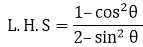Substituting the values from equation (4) and (5), we have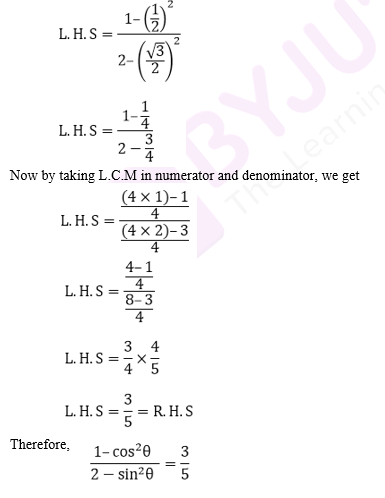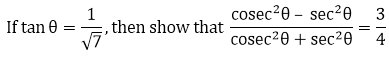16.

Solution:

Given, tan θ = 1/ √7 …..(1)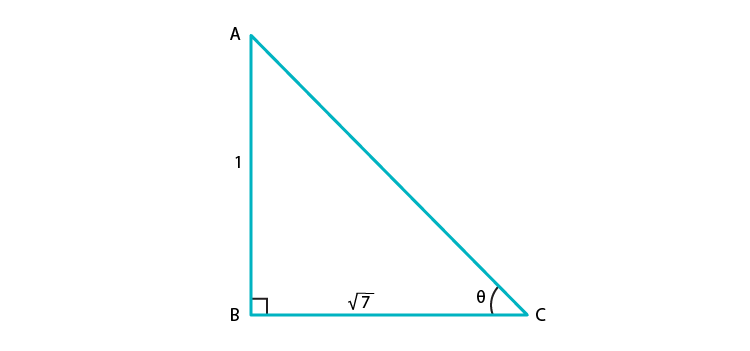By definition, we know that

tan θ = Perpendicular side opposite to ∠θ / Base side adjacent to ∠θ ……(2)

On comparing equations (1) and (2), we have

The perpendicular side opposite to ∠θ = 1

Base side adjacent to ∠θ = √7

Thus, the triangle representing ∠ θ is,

Hypotenuse AC is unknown, and it can be found by using Pythagoras theorem.

By applying Pythagoras theorem, we have

AC= AB2 + BC2

AC= 12 + (√7)2

AC 2 = 1 + 7

AC2 = 8

AC = √8

⇒ AC = 2√2

By definition,

sin θ = Perpendicular side opposite to ∠θ / Hypotenuse = AB / AC

⇒ sin θ = 1/ 2√2

And, since cosec θ = 1/sin θ

⇒ cosec θ = 2√2 …….. (3)

Now,

cos θ = Base side adjacent to ∠θ / Hypotenuse = BC / AC

⇒ cos θ = √7/ 2√2

And, since sec θ = 1/ sin θ

⇒ sec θ = 2√2/ √7 ……. (4)

Taking the L.H.S of the equation,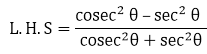Substituting the value of cosec θ and sec θ from equation (3) and (4), we get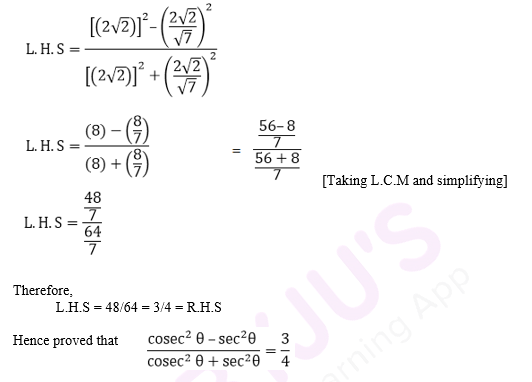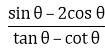17. If sec θ = 5/4, find the value of

Solution:

Given,

sec θ = 5/4

We know that,

sec θ = 1/ cos θ

⇒ cos θ = 1/ (5/4) = 4/5 …… (1)

By definition,

cos θ = Base side adjacent to ∠θ / Hypotenuse …. (2)

On comparing equation (1) and (2), we have

Hypotenuse = 5

Base side adjacent to ∠θ = 4

Thus, the triangle representing ∠ θ is ABC.

Perpendicular side opposite to ∠θ, AB is unknown, and it can be found by using Pythagoras theorem.

By applying Pythagoras’ theorem, we have

AC= AB2 + BC2

AB= AC2 + BC2

AB2 = 52 – 42

AB2 = 25 – 16

AB = √9

⇒ AB = 3

By definition,

sin θ = Perpendicular side opposite to ∠θ / Hypotenuse = AB / AC

⇒ sin θ = 3/ 5 …..(3)

Now, tan θ = Perpendicular side opposite to ∠θ / Base side adjacent to ∠θ

⇒ tan θ = 3/ 4 ……(4)

And, since cot θ = 1/ tan θ

⇒ cot θ = 4/ 3 ……(5)

Now,

Substituting the value of sin θ, cos θ, cot θ and tan θ from the equations (1), (3), (4) and (5), we have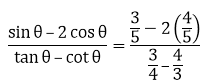= 12/7

Therefore,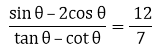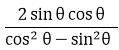18. If tan θ = 12/13, find the value of

Solution:

Given,

tan θ = 12/13 …….. (1)

We know that by definition,

tan θ = Perpendicular side opposite to ∠θ / Base side adjacent to ∠θ …… (2)

On comparing equation (1) and (2), we have

The perpendicular side opposite to ∠θ = 12

Base side adjacent to ∠θ = 13

Thus, in the triangle representing ∠ θ, we have,

Hypotenuse AC is the unknown, and it can be found by using Pythagoras theorem.

So, by applying Pythagoras’ theorem, we have

AC= 122 + 132

AC 2 = 144 + 169

AC2 = 313

⇒ AC = √313

By definition,

sin θ = Perpendicular side opposite to ∠θ / Hypotenuse = AB / AC

⇒ sin θ = 12/ √313…..(3)

And, cos θ = Base side adjacent to ∠θ / Hypotenuse = BC / AC

⇒ cos θ = 13/ √313 …..(4)

Now, substituting the value of sin θ and cos θ from equation (3) and (4), respectively, in the equation below,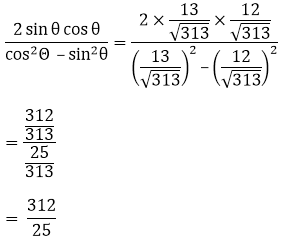Therefore,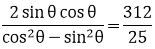### RD Sharma Solutions for Class 10 Maths Chapter 5 Exercise 5.2 Page No: 5.41

Evaluate each of the following:

1. sin 45 sin 30 + cos 45 cos 30

Solution: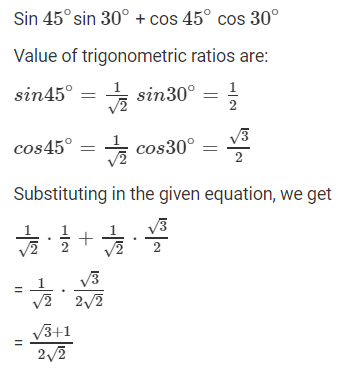2. sin 60 cos 30 + cos 60 sin 30

Solution: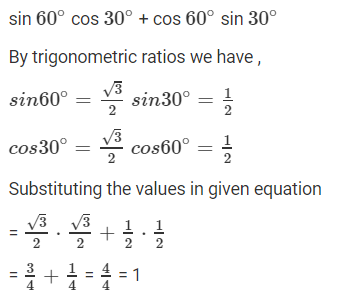3.  cos 60 cos 45 – sin 60 sin 45

Solution: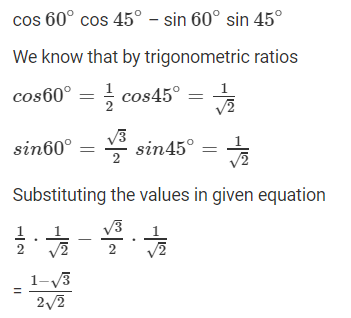4. sin2 30 + sin2 45 + sin2 60 + sin2 90

Solution: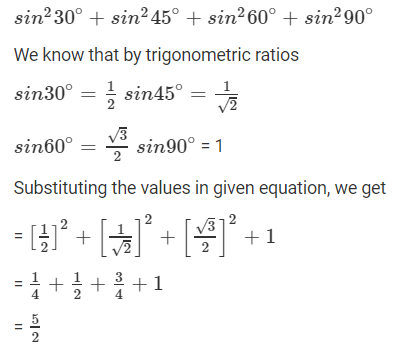5.  cos2 30 + cos2 45 + cos2 60 + cos2 90

Solution: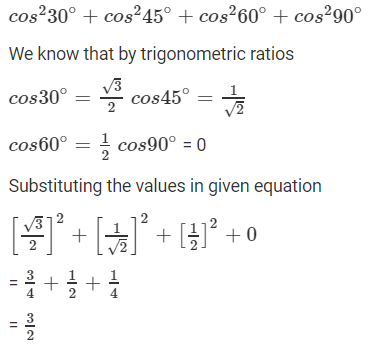6. tan2 30 + tan2 45 + tan2 60

Solution: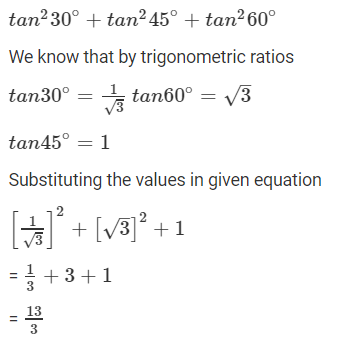7. 2sin2 30 − 3cos2 45 + tan2 60

Solution: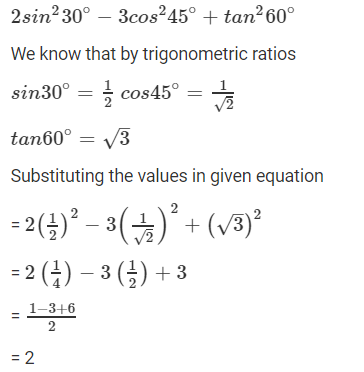8. sin2 30 cos245 + 4tan2 30 + (1/2) sin2 90 − 2cos2 90 + (1/24) cos20

Solution: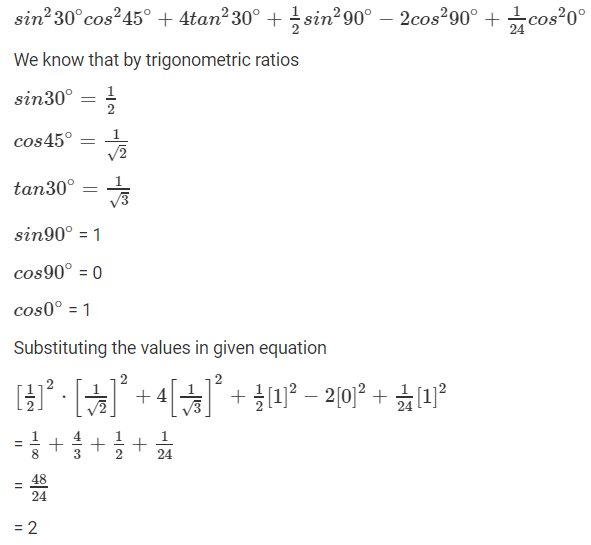9. 4(sin4 60 + cos4 30) − 3(tan2 60− tan2 45) + 5cos2 45

Solution: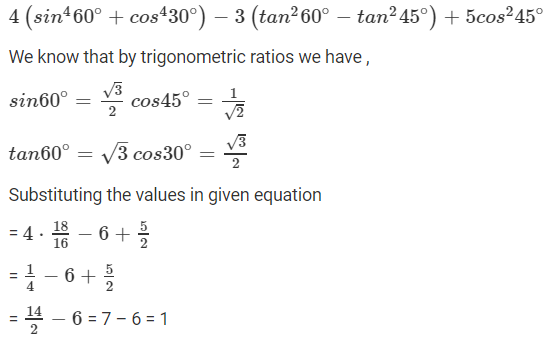10. (cosec2 45 sec2 30)(sin2 30 + 4cot2 45 − sec2 60)

Solution: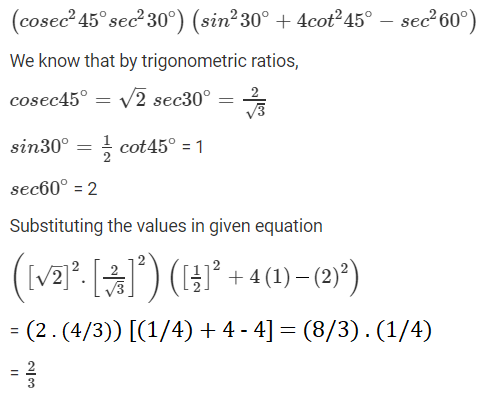11.  cosec3 30 cos60 tan3 45 sin2 90 sec2 45 cot30

Solution: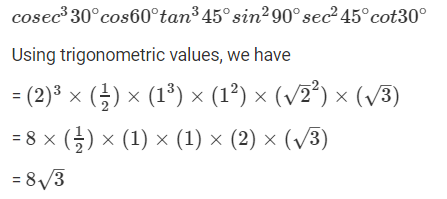12. cot2 30 − 2cos2 60 − (3/4)sec2 45 – 4sec2 30

Solution:

Using trigonometric values, we have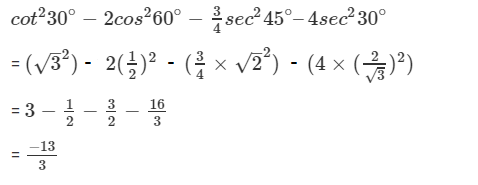13. (cos0 + sin45 + sin30)(sin90 − cos45 + cos60)

Solution:

(cos0 + sin45 + sin30)(sin90 − cos45 + cos60)

Using trigonometric values, we have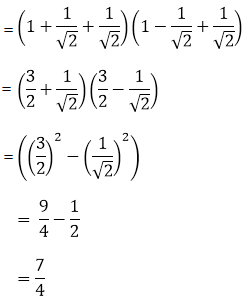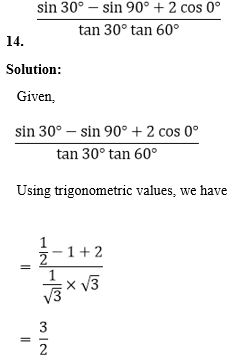15. 4/cot2 30 + 1/sin2 60 − cos2 45

Solution: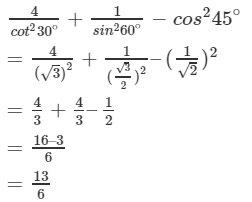16. 4(sin4 30 + cos2 60) − 3(cos2 45 − sin2 90) − sin2 60

Solution:

Using trigonometric values, we have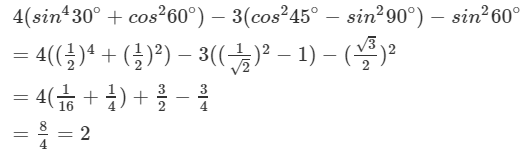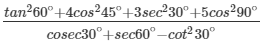17.

Solution:

Using trigonometric values, we have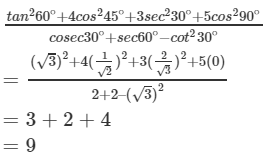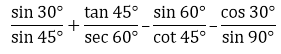18.

Solution:

Using trigonometric values, we have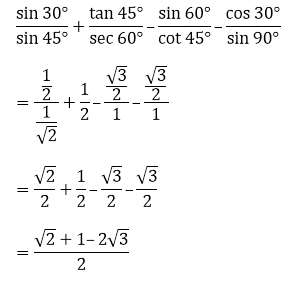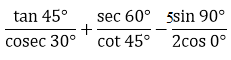19.

Solution:

Using trigonometric values, we have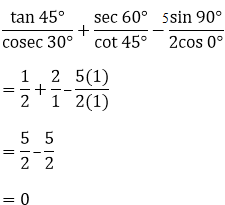Find the value of x in each of the following: (20-25)

20. 2sin 3x = √3

Solution:

Given,

2 sin 3x = √3

sin 3x = √3/2

sin 3x = sin 60°

3x = 60°

x = 20°

### RD Sharma Solutions for Class 10 Maths Chapter 5 Exercise 5.3 Page No: 5.52

1. Evaluate the following:

(i) sin 20o/ cos 70o

(ii) cos 19o/ sin 71o

(iii) sin 21o/ cos 69o

(iv) tan 10o/ cot 80o

(v) sec 11o/ cosec 79o

Solution:

(i) We have,

sin 20o/ cos 70o = sin (90o – 70o)/ cos 70o = cos 70o/ cos70o = 1 [∵ sin (90 – θ) = cos θ]

(ii) We have,

cos 19o/ sin 71o = cos (90o – 71o)/ sin 71o = sin 71o/ sin 71o = 1 [∵ cos (90 – θ) = sin θ]

(iii) We have,

sin 21o/ cos 69o = sin (90o – 69o)/ cos 69o = cos 69o/ cos69o = 1 [∵ sin (90 – θ) = cos θ]

(iv) We have,

tan 10o/ cot 80o = tan (90o – 10o) / cot 80o = cot 80o/ cos80o = 1 [∵ tan (90 – θ) = cot θ]

(v) We have,

sec 11o/ cosec 79o = sec (90o – 79o)/ cosec 79o = cosec 79o/ cosec 79o = 1

[∵ sec (90 – θ) = cosec θ]

2. Evaluate the following: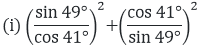Solution:

We have, [∵ sin (90 – θ) = cos θ and cos (90 – θ) = sin θ]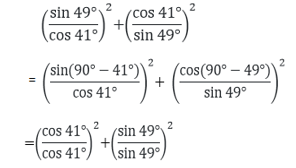= 12 + 12 = 1 + 1

= 2

(ii) cos 48°- sin 42°

Solution:

We know that, cos (90° − θ) = sin θ.

So,

cos 48° – sin 42° = cos (90° − 42°) – sin 42° = sin 42° – sin 42°= 0

Thus, the value of cos 48° – sin 42° is 0.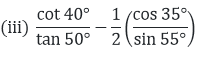Solution:

We have, [∵ cot (90 – θ) = tan θ and cos (90 – θ) = sin θ]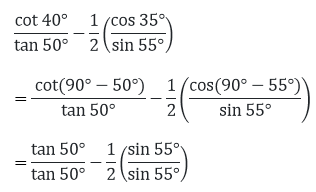= 1 – 1/2(1)

= 1/2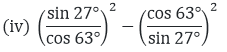Solution:

We have, [∵ sin (90 – θ) = cos θ and cos (90 – θ) = sin θ]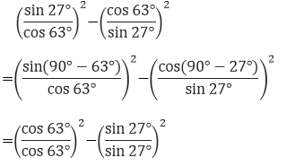= 1 – 1

= 0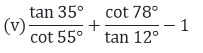Solution:

We have [∵ cot (90 – θ) = tan θ and tan (90 – θ) = cot θ]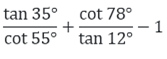= tan (90o – 35o)/ cot 55o + cot (90o – 12o)/ tan 12o – 1

= cot 55o/ cot 55o + tan 12o/ tan 12o – 1

= 1 + 1 – 1

= 1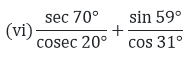Solution:

We have [∵ sin (90 – θ) = cos θ and sec (90 – θ) = cosec θ]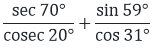= sec (90o – 20o)/ cosec 20o + sin (90o – 31o)/ cos 31o

= cosec 20o/ cosec 20o + cos 12o/ cos 12o

= 1 + 1

= 2

(vii) cosec 31° – sec 59°

Solution:

We have,

cosec 31° – sec 59°

Since, cosec (90 – θ) = cos θ

So,

cosec 31° – sec 59° = cosec (90° – 59o) – sec 59° = sec 59° – sec 59° = 0

Thus,

cosec 31° – sec 59° = 0

(viii) (sin 72° + cos 18°) (sin 72° – cos 18°)

Solution:

We know that,

sin (90 – θ) = cos θ

So, the given can be expressed as

(sin 72° + cos 18°) (sin (90 – 18)° – cos 18°)

= (sin 72° + cos 18°) (cos 18° – cos 18°)

= (sin 72° + cos 18°) x 0

= 0

(ix) sin 35° sin 55° – cos 35° cos 55°

Solution:

We know that,

sin (90 – θ) = cos θ

So, the given can be expressed as

sin (90 – 55)° sin (90 – 35)° – cos 35° cos 55°

= cos 55° cos 35° – cos 35° cos 55°

= 0

(x) tan 48° tan 23° tan 42° tan 67°

Solution:

We know that,

tan (90 – θ) = cot θ

So, the given can be expressed as

tan (90 – 42)° tan (90 – 67)° tan 42° tan 67°

= cot 42° cot 67° tan 42° tan 67°

= (cot 42° tan 42°)(cot 67° tan 67°)

= 1 x 1 [∵ tan θ x cot θ = 1]

= 1

(xi) sec 50° sin 40° + cos 40° cosec 50°

Solution:

We know that,

sin (90 – θ) = cos θ and cos (90 – θ) = sin θ

So, the given can be expressed as

sec 50° sin (90 – 50)° + cos (90 – 50)° cosec 50°

= sec 50° cos 50° + sin 50° cosec 50°

= 1 + 1 [∵ sin θ x cosec θ = 1 and cos θ x sec θ = 1]

= 2

3. Express each one of the following in terms of trigonometric ratios of angles lying between 0o and 45o

(i) sin 59o + cos 56o (ii) tan 65o + cot 49o (iii) sec 76o + cosec 52o

(iv) cos 78o + sec 78o (v) cosec 54o + sin 72o (vi) cot 85o + cos 75o

(vii) sin 67o + cos 75o

Solution:

Using the below trigonometric ratios of complementary angles, we find the required.

sin (90 – θ) = cos θ cosec (90 – θ) = sec θ

cos (90 – θ) = sin θ sec (90 – θ) = cosec θ

tan (90 – θ) = cot θ cot (90 – θ) = tan θ

(i) sin 59o + cos 56o = sin (90 – 31)o + cos (90 – 34)o = cos 31o + sin 34o

(ii) tan 65o + cot 49o = tan (90 – 25)o + cot (90 -41)o = cot 25o + tan 41o

(iii) sec 76o + cosec 52o = sec (90 – 14)o + cosec (90 – 38)o = cosec 14o + sec 38o

(iv) cos 78o + sec 78o = cos (90 – 12)o + sec (90 – 12)o = sin 12o + cosec 12o

(v) cosec 54o + sin 72o = cosec (90 – 36)o + sin (90 – 18)o = sec 36o + cos 18o

(vi) cot 85o + cos 75o = cot (90 – 5)o + cos (90 – 15)o = tan 5o + sin 15o

4. Express cos 75o + cot 75o in terms of angles between 0o and 30o.

Solution:

Given,

cos 75o + cot 75o

Since, cos (90 – θ) = sin θ and cot (90 – θ) = tan θ

cos 75o + cot 75o = cos (90 – 15)o + cot (90 – 15)o = sin 15o + tan 15o

Hence, cos 75o + cot 75o can be expressed as sin 15o + tan 15o

5. If sin 3A = cos (A – 26o), where 3A is an acute angle, find the value of A.

Solution:

Given,

sin 3A = cos (A – 26o)

Using cos (90 – θ) = sin θ, we have

sin 3A = sin (90o – (A – 26o))

Now, comparing both L.H.S. and R.H.S.,

3A = 90o – (A – 26o)

3A + (A – 26o) = 90o

4A – 26o = 90o

4A = 116o

A = 116o/4

∴ A = 29o

6. If A, B, and C are the interior angles of a triangle ABC, prove that

(i) tan ((C + A)/ 2) = cot (B/2) (ii) sin ((B + C)/ 2) = cos (A/2)

Solution:

We know that, in triangle ABC the sum of the angles, i.e., A + B + C = 180o

So, C + A = 180o – B ⇒ (C + A)/2 = 90o – B/2 …… (i)

And, B + C = 180o – A ⇒ (B + C)/2 = 90o – A/2 ……. (ii)

(i) L.H.S = tan ((C + A)/ 2)

⇒ tan ((C + A)/ 2) = tan (90o – B/2) [From (i)]

= cot (B/2) [∵ tan (90 – θ) = cot θ]

= R.H.S

• Hence, proved

(ii) L.H.S = sin ((B + C)/2)

⇒ sin ((B + C)/ 2) = sin (90o – A/2) [From (ii)]

= cos (A/2)

= R.H.S.

• Hence, proved

7. Prove that:

(i) tan 20° tan 35° tan 45° tan 55° tan 70° = 1

(ii) sin 48° sec 48° + cos 48° cosec 42° = 2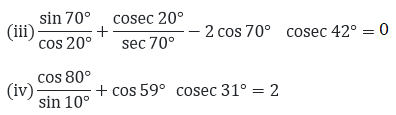Solution:

(i) Taking L.H.S = tan 20° tan 35° tan 45° tan 55° tan 70°

= tan (90° − 70°) tan (90° − 55°) tan 45°tan 55° tan70°

= cot 70°cot 55° tan 45° tan 55° tan 70°  [∵ tan (90 – θ) = cot θ]

= (tan 70°cot 70°)(tan 55°cot 55°) tan 45°  [∵ tan θ x cot θ = 1]

= 1 × 1 × 1 = 1

• Hence, proved

(ii) Taking L.H.S = sin 48° sec 48° + cos 48° cosec 42°

= sin 48° sec (90° − 48°) + cos 48° cosec (90° − 48°)

[∵sec (90 – θ) = cosec θ and cosec (90 – θ) = sec θ]

= sin 48°cosec 48° + cos 48°sec 48°  [∵ cosec θ x sin θ = 1 and cos θ x sec θ = 1]

= 1 + 1 = 2

• Hence, proved

(iii) Taking the L.H.S,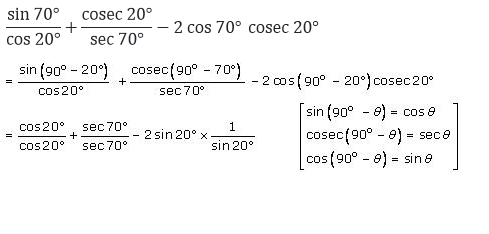= 1 + 1 – 2

= 2 – 2

= 0

• Hence, proved

(iv) Taking L.H.S,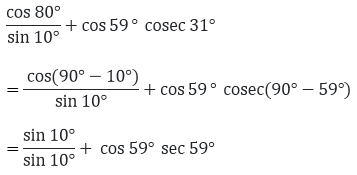= 1 + 1

= 2

• Hence, proved

8. Prove the following:

(i) sinθ sin (90o – θ) – cos θ cos (90o – θ) = 0

Solution:

Taking the L.H.S,

sinθ sin (90o – θ) – cos θ cos (90o – θ)

= sin θ cos θ – cos θ sin θ [∵ sin (90 – θ) = cos θ and cos (90 – θ) = sin θ]

= 0

(ii)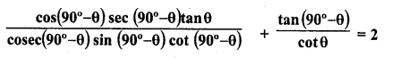Solution:

Taking the L.H.S,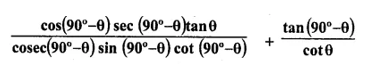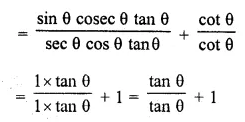[∵ cosec θ x sin θ = 1 and cos θ x sec θ = 1]

= 1 + 1

= 2 = R.H.S

• Hence, proved

(iii)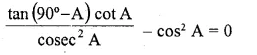Solution:

Taking the L.H.S., [∵ tan (90o – θ) = cot θ]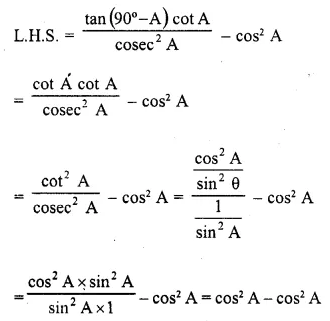= 0 = R.H.S.

• Hence, proved

(iv)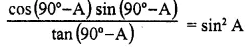Solution:

Taking L.H.S., [∵ sin (90 – θ) = cos θ and cos (90 – θ) = sin θ]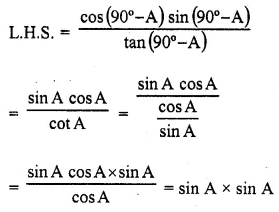= sin2 A = R.H.S.

• Hence, proved

(v) sin (50o + θ) – cos (40oθ) + tan 1o tan 10o tan 20o tan 70o tan 80o tan 89o = 1

Solution:

Taking the L.H.S.,

= sin (50o + θ) – cos (40o – θ) + tan 1o tan 10o tan 20o tan 70o tan 80o tan 89o

= [sin (90o – (40o – θ))] – cos (40o – θ) + tan (90 – 89)o tan (90 – 80)o tan (90 – 70)o tan 70o tan 80o tan 89o [∵ sin (90 – θ) = cos θ]

= cos (40o – θ) – cos (40o – θ) + cot 89o cot 80o cot 70o tan 70o tan 80o tan 89o

[∵ tan (90o – θ) = cot θ]

= 0 + (cot 89o x tan 89o) (cot 80o x tan 80o) (cot 70o x tan 70o)

= 0 + 1 x 1 x 1 [∵ tan θ x cot θ = 1]

= 1= R.H.S

• Hence, proved

## Frequently Asked Questions on RD Sharma Solutions for Class 10 Maths Chapter 5

Q1

### Why should we learn all the concepts present in RD Sharma Solutions for Class 10 Maths Chapter 5?

The concepts covered in Chapter 5 of RD Sharma Solutions Maths provide important questions based on the exam pattern and model question paper. So it is necessary to learn all the concepts present in RD Sharma Solutions for Class 10 Maths Chapter 5. Our subject experts prepared these exercises to help students with their exam preparation to achieve good marks in Maths. The solutions are developed in a simple and lucid manner to boost learning skills among students.
Q2

### Name the important topic students learn in RD Sharma Solutions for Class 10 Maths Chapter 5.

Students learn about Trigonometric ratios and their relations between them in the RD Sharma Solutions for Class 10 Maths Chapter 5. Trigonometry is the science of measuring triangles. The trigonometric ratios of some specific angles are discussed in this chapter for better understanding among students. These concepts are important from an exam perspective. It is strictly based on the latest syllabus of the CBSE board and also depends on the CBSE question paper design and marking scheme.
Q3

### How many exercises are there in RD Sharma Solutions for Class 10 Maths Chapter 5?

There are 3 exercises in RD Sharma Solutions for Class 10 Maths Chapter 5. The first exercise contains 18 questions with sub questions, the second exercise contains 20 questions with sub questions, and the third exercise has 8 questions with sub questions based on the concepts of trigonometric ratios. The solutions to all questions in RD Sharma books are given here in a precise manner to help the students understand the concepts more effectively.